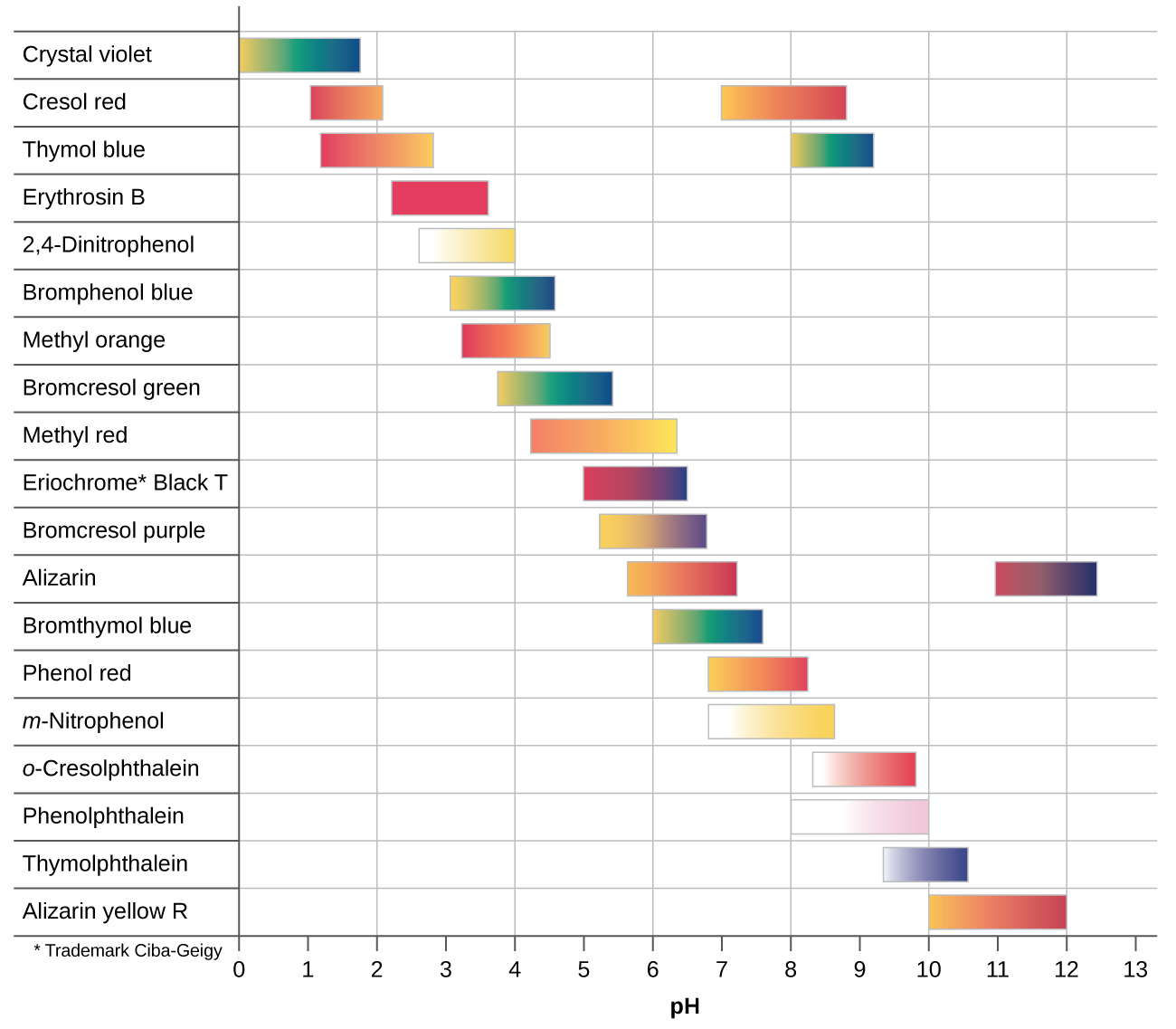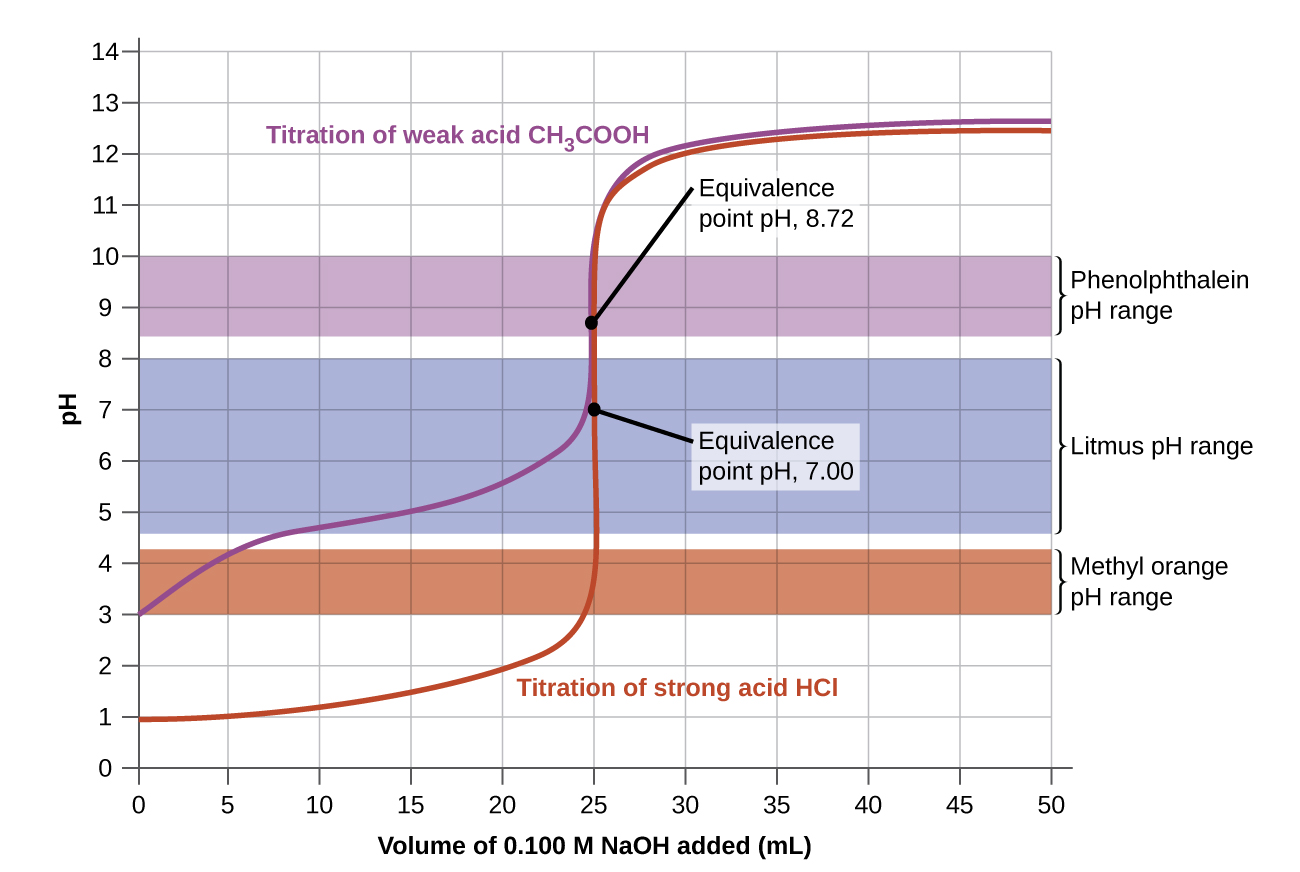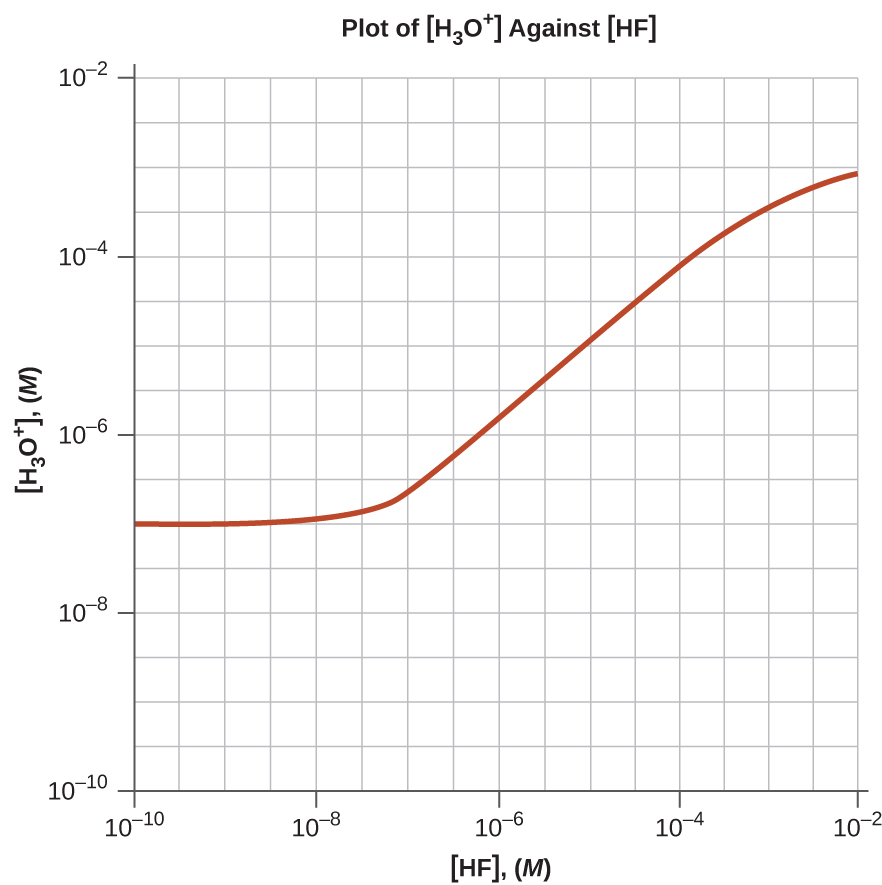# 14.7 Acid-base titrations  (Page 5/8)

 Page 5 / 8
$\frac{\left[{\text{H}}_{3}{\text{O}}^{\text{+}}\right]}{{K}_{\text{a}}}\phantom{\rule{0.2em}{0ex}}=\phantom{\rule{0.2em}{0ex}}\frac{\left[\text{HIn}\right]}{\left[{\text{In}}^{\text{−}}\right]}$
$\text{log}\left(\phantom{\rule{0.2em}{0ex}}\frac{\left[{\text{H}}_{3}{\text{O}}^{\text{+}}\right]}{{K}_{\text{a}}}\right)\phantom{\rule{0.2em}{0ex}}\phantom{\rule{0.2em}{0ex}}=\text{log}\left(\phantom{\rule{0.2em}{0ex}}\frac{\left[\text{HIn}\right]}{\left[{\text{In}}^{\text{−}}\right]}\right)\phantom{\rule{0.2em}{0ex}}$
$\text{log}\left(\left[{\text{H}}_{3}{\text{O}}^{\text{+}}\right]\right)-\text{log}\left({K}_{\text{a}}\right)=\text{−log}\left(\phantom{\rule{0.2em}{0ex}}\frac{\left[{\text{In}}^{\text{−}}\right]}{\left[\text{HIn}\right]}\right)\phantom{\rule{0.2em}{0ex}}$
$-\text{pH}+\text{p}{K}_{\text{a}}=\text{−log}\left(\phantom{\rule{0.2em}{0ex}}\frac{\left[{\text{In}}^{\text{−}}\right]}{\left[\text{HIn}\right]}\right)\phantom{\rule{0.2em}{0ex}}$
$\text{pH}=\text{p}K\text{a}+\text{log}\left(\phantom{\rule{0.2em}{0ex}}\frac{\left[{\text{In}}^{\text{−}}\right]}{\left[\text{HIn}\right]}\right)\phantom{\rule{0.4em}{0ex}}\text{or}\phantom{\rule{0.3em}{0ex}}\text{pH}=\text{p}{K}_{\text{a}}+\text{log}\left(\phantom{\rule{0.2em}{0ex}}\frac{\left[\text{base}\right]}{\left[\text{acid}\right]}\right)\phantom{\rule{0.2em}{0ex}}$

The last formula is the same as the Henderson-Hasselbalch equation, which can be used to describe the equilibrium of indicators.

When [H 3 O + ] has the same numerical value as K a , the ratio of [In ] to [HIn]is equal to 1, meaning that 50% of the indicator is present in the red form (HIn) and 50% is in the yellow ionic form (In ), and the solution appears orange in color. When the hydronium ion concentration increases to 8 $×$ 10 −4 M (a pH of 3.1), the solution turns red. No change in color is visible for any further increase in the hydronium ion concentration (decrease in pH). At a hydronium ion concentration of 4 $×$ 10 −5 M (a pH of 4.4), most of the indicator is in the yellow ionic form, and a further decrease in the hydronium ion concentration (increase in pH) does not produce a visible color change. The pH range between 3.1 (red) and 4.4 (yellow) is the color-change interval    of methyl orange; the pronounced color change takes place between these pH values.

There are many different acid-base indicators that cover a wide range of pH values and can be used to determine the approximate pH of an unknown solution by a process of elimination. Universal indicators and pH paper contain a mixture of indicators and exhibit different colors at different pHs. [link] presents several indicators, their colors, and their color-change intervals.This chart illustrates the ranges of color change for several acid-base indicators.

Titration curves help us pick an indicator that will provide a sharp color change at the equivalence point. The best selection would be an indicator that has a color change interval that brackets the pH at the equivalence point of the titration.

The color change intervals of three indicators are shown in [link] . The equivalence points of both the titration of the strong acid and of the weak acid are located in the color-change interval of phenolphthalein. We can use it for titrations of either strong acid with strong base or weak acid with strong base.The graph shows a titration curve for the titration of 25.00 mL of 0.100 M CH 3 CO 2 H (weak acid) with 0.100 M NaOH (strong base) and the titration curve for the titration of HCl (strong acid) with NaOH (strong base). The pH ranges for the color change of phenolphthalein, litmus, and methyl orange are indicated by the shaded areas.

Litmus is a suitable indicator for the HCl titration because its color change brackets the equivalence point. However, we should not use litmus for the CH 3 CO 2 H titration because the pH is within the color-change interval of litmus when only about 12 mL of NaOH has been added, and it does not leave the range until 25 mL has been added. The color change would be very gradual, taking place during the addition of 13 mL of NaOH, making litmus useless as an indicator of the equivalence point.

We could use methyl orange for the HCl titration, but it would not give very accurate results: (1) It completes its color change slightly before the equivalence point is reached (but very close to it, so this is not too serious); (2) it changes color, as [link] shows, during the addition of nearly 0.5 mL of NaOH, which is not so sharp a color change as that of litmus or phenolphthalein; and (3) it goes from yellow to orange to red, making detection of a precise endpoint much more challenging than the colorless to pink change of phenolphthalein. [link] shows us that methyl orange would be completely useless as an indicator for the CH 3 CO 2 H titration. Its color change begins after about 1 mL of NaOH has been added and ends when about 8 mL has been added. The color change is completed long before the equivalence point (which occurs when 25.0 mL of NaOH has been added) is reached and hence provides no indication of the equivalence point.

We base our choice of indicator on a calculated pH, the pH at the equivalence point. At the equivalence point, equimolar amounts of acid and base have been mixed, and the calculation becomes that of the pH of a solution of the salt resulting from the titration.

## Key concepts and summary

A titration curve is a graph that relates the change in pH of an acidic or basic solution to the volume of added titrant. The characteristics of the titration curve are dependent on the specific solutions being titrated. The pH of the solution at the equivalence point may be greater than, equal to, or less than 7.00. The choice of an indicator for a given titration depends on the expected pH at the equivalence point of the titration, and the range of the color change of the indicator.

Explain how to choose the appropriate acid-base indicator for the titration of a weak base with a strong acid.

At the equivalence point in the titration of a weak base with a strong acid, the resulting solution is slightly acidic due to the presence of the conjugate acid. Thus, pick an indicator that changes color in the acidic range and brackets the pH at the equivalence point. Methyl orange is a good example.

Explain why an acid-base indicator changes color over a range of pH values rather than at a specific pH.

Why can we ignore the contribution of water to the concentrations of H 3 O + in the solutions of following acids:

0.0092 M HClO, a weak acid

0.0810 M HCN, a weak acid

0.120 M $\text{Fe}{\left({\text{H}}_{2}\text{O}\right)}_{6}{}^{2+}$ a weak acid, K a = 1.6 $×$ 10 −7

but not the contribution of water to the concentration of OH ?

In an acid solution, the only source of OH ions is water. We use K w to calculate the concentration. If the contribution from water was neglected, the concentration of OH would be zero.

We can ignore the contribution of water to the concentration of OH in a solution of the following bases:

0.0784 M C 6 H 5 NH 2 , a weak base

0.11 M (CH 3 ) 3 N, a weak base

but not the contribution of water to the concentration of H 3 O + ?

Draw a curve for a series of solutions of HF. Plot [H 3 O + ] total on the vertical axis and the total concentration of HF (the sum of the concentrations of both the ionized and nonionized HF molecules) on the horizontal axis. Let the total concentration of HF vary from 1 $×$ 10 −10 M to 1 $×$ 10 −2 M .Draw a curve similar to that shown in [link] for a series of solutions of NH 3 . Plot [OH ] on the vertical axis and the total concentration of NH 3 (both ionized and nonionized NH 3 molecules) on the horizontal axis. Let the total concentration of NH 3 vary from 1 $×$ 10 −10 M to 1 $×$ 10 −2 M .

Calculate the pH at the following points in a titration of 40 mL (0.040 L) of 0.100 M barbituric acid ( K a = 9.8 $×$ 10 −5 ) with 0.100 M KOH.

(b) 20 mL of KOH solution added

(c) 39 mL of KOH solution added

(d) 40 mL of KOH solution added

(e) 41 mL of KOH solution added

(a) pH = 2.50;
(b) pH = 4.01;
(c) pH = 5.60;
(d) pH = 8.35;
(e) pH = 11.08

The indicator dinitrophenol is an acid with a K a of 1.1 $×$ 10 −4 . In a 1.0 $×$ 10 −4 - M solution, it is colorless in acid and yellow in base. Calculate the pH range over which it goes from 10% ionized (colorless) to 90% ionized (yellow).

given that 32.og sulphur contains 6.02×10 ^23 sulphur atoms how many atoms are there in 2.70g of aluminum. (A=27 s =32 )
0.602*10^23=6.02×10^22
galina
Correct 👍... Its 1/10 of the mass number of Al which is 27
Karim
show the steps pls
Faith
correct
Collins
2.7g of Al - 6.02×10^23×2.7/27=0.602×10^23
galina
thanks
Faith
An aqueos solution of sodium hydroxide containing 2g in 200cm3 of solution has a concentration of (NaOH=40).
0.25 mol/dm3
David
0.25M
David
what is the meaning of the term an orbital?
Orbital mathematical function that describes the wave-like behavior of an electron, electron pair, or (less commonly) nucleons. An orbital may also be called an atomic orbital or electron
Emmanuel
orbitals describe where an electron is likely to be found in an atom. Molecular orbitals perform the same role in molecules
Danny
why is alkynes more reactive than alkenes and alkanes
Alkenes and alkynes are generally more reactive than alkanes due to the electron density available in their pi bonds.
Danny
What are molecules
a group of atoms bonded together, representing the smallest fundamental unit of a chemical compound that can take part in a chemical reaction
Danny
True
David
exactly
Harriet
true
lucy
were is ruth
Danny
Perfect
BISWAJIT
what is partial pressure law
what is the structural formular for 2,3-dimethylbutane?
name the following compound (COOH)2
Mercy
CH3-CH(CH3)-CH(CH3)-CH3 this is 2,3-dimethylbutane
galina
(COOH)2 is oxalic acid
thiru
what is chemistry
chemistry is the study of chemical reactions
lucy
Chemistry is the study of matter, its properties, how and why substances combine or separate to form other substances, and how substances interact with energy.
Danny
chemistry is the study of matter, it's properties and composition
alexandra
am from Abuja and am in ss1
lucy
ss1 some one translate that for me we use grades in my country
Danny
lucy
okay am a chemistry and biology teacher from Zambia
Danny
should be grade 10 I think
alexandra
thats good Mr mwanza
Harriet
Danny
GCE 12
Harriet
lusaka
Harriet
yes sir
lucy
me ss2
Richard
so are a final year student
kabu
What is the chemical formula for alcohol? is the chemical formula for alcohol?
CH3CH2OH
Anupam
20 element are what element?
What is Chemistry?
thiru
Esther
studying about the rate of chemical is ?
thiru
Chemistry is the branch of sciences dealing with the composition of substances and their properties and reaction.
Jeremiah
chemical reaction is the process in which one or more chemical substances react with other chemical substance and converted one or more different substances.
Aisha
what is isotopy
Isotopy is one of two or more atoms having the same atomic numbers but different mass number.
Esther
is phenomenon where by atom of an element exhibit different mass number but have the same atomic number
lucy
same number of electrons and protons but different number of neutrons
galina
is an atom which has different number of protons but same number of electron such as carbon -12 and corbon 12
Collins
carbon-12 and carbon-13 have 6 electrons and 6 protons but carbon-12 has 6 neutrons and carbon-13 has 7 neutrons. Isotopes have different amount of neutrons
galina
Molar mass of Chlorine
molar mass of chlorine 35.5
lucy
what is chemistry
Molar mass of Chlorine atom
Sakyi
chem is the study of structure, properties of matter and changes that matter undergo
Sheila
m.m of Cl is 35.5
Sheila
SYOPI chemistry is the study of changes in the study of matter
syopi
what is an acid
example, HCl, H2SO4, HNO3
galina
an acid is a substance that dissolves in water to give hydrogen oins as the only negatively charged ions
Sheila
Galina is correct those are some examples of acids , others are ethanedioic acid, ethanoic acid , methanoic acid ma ans above is tha definition of an acid
Sheila
An acid is a substance with particular chemical properties including litmus red, neutralizing alkalis and dissolving some metals.
Esther
Shelia is also right 💯💯
Esther
what is hydrocarbon?
Edgar
is a group of carbon atoms that is bonded with an alkyl group eg methyl butane
Sheila
A hydrocarbon is a compound that contains only hydrogen and carbon atoms
martha
it means only carbon and hydrogen atoms are bonded..
EdgarByBy RhodesBy Kimberly NicholsByBy OpenStaxBy Rohini AjayBy Tod McGrathBy OpenStaxBy Anh DaoBy Stephen VoronBy David Corey